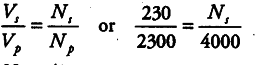# A power transmission line feeds input power at 2300 V

A power transmission line feeds input power at 2300 V to a step-down transformer with its primary windings having 4000 turns. What should be the number of turns in the secondary in order to get output power at 230 V?

Given primary voltage, { V}_{ p } = 2300 V
secondary voltage, { V}_{ s } = 230 V
Primary turns { N}_{ p } = 4000 turns
Here, we assume that the transformer is ideal.No power loss in the form of heat,etc.
Using the formula,{ N}_{ s } = 400